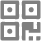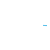Air Jordan 11 Full Red come with real Carbon Fiber, size 41-47 ( 41=8 42=8.5 43=9.5 44=10 45=11 46=12 47=13)

# ISUPLY SNEAKER WA: +86 18965578831

Home
album
Contact
QR code## Air Jordan 11 Full Red come with real Carbon Fiber, size 41-47 ( 41=8 42=8.5 43=9.5 44=10 45=11 46=12 47=13)11

Air Jordan 11 Full Red come with real Carbon Fiber, size 41-47 ( 41=8 42=8.5 43=9.5 44=10 45=11 46=12 47=13) In order to know price or order, please contact me Email: shtradetony@hotmail.com SKPYE ID: SHTRADE.TONY

#### WeChat scan codeOther ways to share### Air Jordan 11 Full Red come with real Carbon Fiber, size 41-47 ( 41=8 42=8.5 43=9.5 44=10 45=11 46=12 47=13)### Air Jordan 11 Full Red come with real Carbon Fiber, size 41-47 ( 41=8 42=8.5 43=9.5 44=10 45=11 46=12 47=13)### Air Jordan 11 Full Red come with real Carbon Fiber, size 41-47 ( 41=8 42=8.5 43=9.5 44=10 45=11 46=12 47=13)### Air Jordan 11 Full Red come with real Carbon Fiber, size 41-47 ( 41=8 42=8.5 43=9.5 44=10 45=11 46=12 47=13)### Air Jordan 11 Full Red come with real Carbon Fiber, size 41-47 ( 41=8 42=8.5 43=9.5 44=10 45=11 46=12 47=13)### Air Jordan 11 Full Red come with real Carbon Fiber, size 41-47 ( 41=8 42=8.5 43=9.5 44=10 45=11 46=12 47=13)### Air Jordan 11 Full Red come with real Carbon Fiber, size 41-47 ( 41=8 42=8.5 43=9.5 44=10 45=11 46=12 47=13)### Air Jordan 11 Full Red come with real Carbon Fiber, size 41-47 ( 41=8 42=8.5 43=9.5 44=10 45=11 46=12 47=13)### Air Jordan 11 Full Red come with real Carbon Fiber, size 41-47 ( 41=8 42=8.5 43=9.5 44=10 45=11 46=12 47=13)### Air Jordan 11 Full Red come with real Carbon Fiber, size 41-47 ( 41=8 42=8.5 43=9.5 44=10 45=11 46=12 47=13)### Air Jordan 11 Full Red come with real Carbon Fiber, size 41-47 ( 41=8 42=8.5 43=9.5 44=10 45=11 46=12 47=13)#### WeChat scan codeOther ways to share